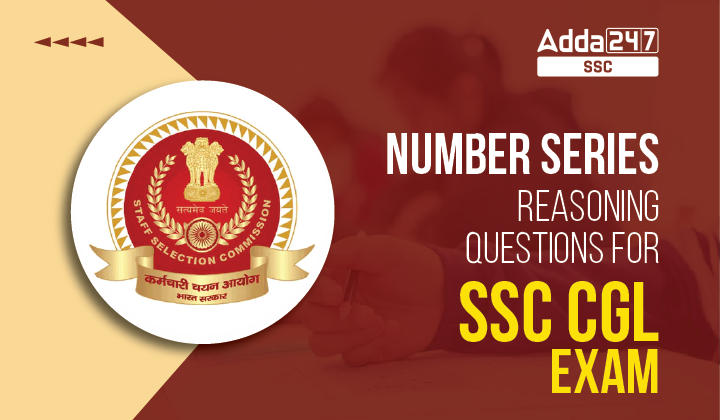Latest SSC jobs   »   Reasoning Questions Quiz   »   Number Series

# Number Series: Reasoning Questions for SSC CGL ExamWe have provided Number Series of Reasoning quiz in accordance with the syllabus of SSC CGL Tier-I. This quiz will help you in getting more marks in the final exam.

Directions(1-2): In each of the following questions a number series is given. Below the series, a number along with (1), (2), (3) (4) and (5) is given. You have to complete the series following the same sequence as that of the given series. Then answer the question that follows.

Q1. 5 , 7,  10,  36, 136,  690
2 ,(1), (2) , (3),  (4),  (5)
What will come in place of (3)?
(a) 26
(b) 64
(c) 18
(d) 46
Ans.(c)
Sol.  The series is based on the following pattern:
5 × 1 + 2 = 7
7 × 2 – 4 = 10
10 × 3 + 6 = 36
36 × 4 – 8 = 136
136 × 5 + 10 = 690
Similarly, the new series is as follows:
2 × 1 + 2 = 4 …. (1)
4 × 2 – 4 = 4 …. (2)
4 × 3 + 6 = 18 …. (3)
Therefore, the number 18 will come in place of (3).

Q2. 8,    4,    6,   15,   52.5,   236.25
4,  (1),  (2), (3),    (4),      (5)
What will come in place of (4)?
(a) 36.25
(b) 26.25
(c) 16.25
(d) 32.75
Ans.(b)
Sol.  The series is based on following pattern:
8 × 0.5 = 4,
4 × 1.5 = 6,
6 × 2.5 = 15,
15 × 3.5 = 52.6,
52.5 × 4.5 = 236.25
Similarly,
4 × 0.5 = 2 …. (1)
2 × 1.5 = 3 …. (2)
3 × 2.5 = 7.5 …. (3)
7.5 × 3.5 = 26.25 . …(4)
Therefore, the number 26.25 will come in place of (4).

Directions(3-5): In each of the following questions a number is missing. Find this missing number from the following alternatives.

Q3. 512, 49, 216, 25, ?
(a) 8
(b) 27
(c) 64
(d) 36
Ans.(c)
Sol.

512,     49,      216,     25,         64
(83),   (72),    (63),     (52),      (43)

Q4. 15, 24, 42, 69, (?), 150
(a) 114
(b) 105
(c) 100
(d) 140
S4. Ans.(b)
Sol.  The pattern of the number series is as given below:
15 + 9 = 24
24 + 18 (= 9 + 9) = 42
42 + 27 (= 18 + 9) = 69
69 + 36 (= 27 + 9) = 105
105 + 45 (= 36 + 9) = 150

Q5. 3, 7, 16, 32, ?, 93
(a) 53
(b) 57
(c) 54
(d) 60
Ans.(b)
Sol.
= 32 + 52
= 32 + 25 = 57
Directions(6-10): In these questions, which of the following number complete the given sequence?

Q6.  8, 24, 12, 36, 18, 54, (….)
(a) 27
(b) 108
(c) 68
(d) 72
Ans. (a)
Sol. Numbers are alternatively multiplied by 3 and divided by 2.
So, the next number = 54 ÷ 2 = 27.

Q7.  2, 6, 12, 20, 30, 42, 56, (….)
(a) 61
(b) 64
(c) 72
(d) 70
Ans.(c)
Sol. The pattern is 1 x 2, 2 x 3, 3 x 4, 4 x 5, 5 x 6, 6 x 7, 7 x 8.
So, the next number is 8 x 9 = 72.

Q8.  4, -8, 16, -32, 64, (….)
(a) 128
(b) -128
(c) 192
(d) -192
Ans.(b)
Sol. Each number is the proceeding number multiplied by -2.
So, the required number is -128.

Q9. 122 , 170 , 290 , ?
(a) 369
(b) 255
(c) 432
(d) 362
Ans.(d)
Sol.  (11)2 = 121+1= 122,
(13)2= 169+1= 170
(17)2= 289 + 1= 290,
Similarly
(19)2 = 361 + 1 = 362

Q10. 2 ,3 , 8 , 63, ?
(a) 3900
(b) 3636
(c) 3968
(d) 3988
Ans.(c)
Sol. 22= 4-1= 3
32= 9-1 = 8
82= 64-1= 63
632=3969-1 = 3968

Direction (11-13): In each of these questions, a number series is given. In each series, only one number is wrong. Find out the wrong number from the given alternatives?

Q11. 32, 16, 24, 65, 210, 945, 5197.5
(a) 16
(b) 210
(c) 65
(d)5197.5
Ans.(c)
Sol.
So, wrong number is 65, it must be replaced by 60.

Q12. 6, 91, 584, 2935, 11756, 35277, 70558
(a) 35277
(b) 584
(c) 2935
(d) 91
Ans.(b)
Sol.  The pattern of number series is as follows:
6 × 7 + 72 = 42 + 49 = 91
91 × 6 + 62 = 546 + 36 = 582  
582 × 5 + 52 = 2910 + 25 = 2935
2935 × 4 + 42 = 11740 + 16 = 11756
11756 × 3 + 32 = 35268 + 9 = 35277
So, wrong number is 584, it must be replaced by 582.

Q13. 3601, 3602, 1803, 604, 154, 36, 12
(a) 3601
(b) 154
(c) 604
(d) 1803
Ans.(b)
Sol.
So, wrong number is 154, it must be replaced by 155.

Directions(14-15): What should come in place of question mark (?) in the following number series?

Q14. 656, 352, 200, 124, 86, (?)
(a) 97
(b) 49
(c) 67
(d) 57
Ans.(c)
Sol.  The pattern of the number series is as given below:
Missing term is 67.

Q15. 4.5, 18,  2.25,  ? , 1.6875, 33.75
(a) 35
(b) 25.5
(c) 27
(d) 43
Ans.(c)
Sol.  The series is
× 4, ÷ 8, × 12, ÷ 16, × 20

## FAQs

### How many questions are asked in the Reasoning section of SSC CGL Tier 1 Exam?

25 Questions for 50 Marks are asked in the Reasoning section of SSC CGL Tier 1 Exam.